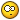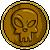# Let's Creat A Group For Writing New Big Program

8 replies to this topic

### #1hazhiriq

hazhiriq

Newbie

•• Members
•• 23 posts
• Interests:Electrical engineering<br />Communication

• Calculators:

Posted 18 January 2008 - 09:40 PM

Hi every one today i see Ti : it have something like Pspice for electrical eng it is so good but we should try to improve our classpad to be more flexible i said this to vanhoa we should creat a group to write a new program because some program like this
http://www.noatechno...4_examples.html
can not be wrote by one it needs a group to do this lets creat a group for writing a biger program I know I am not a very good programer like Vanhoa and .... but if we collect our idea we can improve the classpad.

### #2betoe

betoe

UCF Spanish Translator

•• [Legends]
•• 846 posts
• Gender:Male
• Interests:Electronics, SW development, automotive. Swim->bike->run

• Calculators:

Posted 19 January 2008 - 04:35 AM

Do you have an idea of how to implement something like Pspice?

### #3hazhiriq

hazhiriq

Newbie

•• Members
•• 23 posts
• Interests:Electrical engineering<br />Communication

• Calculators:

Posted 19 January 2008 - 09:02 AM

Yes in the course of electronic physics we study that how the pspice or any program like this analysis the circuite they use an unlinier equation.

### #4far2055

far2055

•• Members
•• 67 posts
• Gender:Male
• Location:Iran
• Interests:Robotics - Programming - Mathematics - Physics - Computer <br />ANN ( Artificial Neural Network )<br />QBasic Lover-->before-->now-&gt;*CPLua&quot; Lover.

• Calculators:

Posted 19 January 2008 - 12:28 PM

It is a good idea to make a group for writing programs. But making some programs like circuit4 isn't so worthy(its engine is not complicated, just the interface is a little difficult). I think we need a technical programming language like scilab or matlab.
We have CPLUA(thanks to Orwell), CPLUA is really a revolution in classpad. We need programs like that. CPLUA is a good programming language but it is a general purpose not a technical language. I know it is really difficult to do specially if we decide to add algebra to it. Our classpad algebra is not good enough(I don't mean it is weak for a calculator), specially some functions aren't able to operate on matrixes(I think it was a big mistake by Casio not to making the CAS base on matrix operation.)
I think making a group to port a CAS like GiNaC and building a technical language is really better than making any other programs.

### #5far2055

far2055

•• Members
•• 67 posts
• Gender:Male
• Location:Iran
• Interests:Robotics - Programming - Mathematics - Physics - Computer <br />ANN ( Artificial Neural Network )<br />QBasic Lover-->before-->now-&gt;*CPLua&quot; Lover.

• Calculators:

Posted 19 January 2008 - 01:05 PM

Yes in the course of electronic physics we study that how the pspice or any program like this analysis the circuite they use an unlinier equation.

By using the laplace transforms or fourier transforms it is not really difficult to analysis a circuit. I study the examples, they were really simple and just taking some minutes to analyse.
check this site. Circuit4 use Node Analysis technique. very very simple to do.
http://www.utwired.e...d1/lesson06.cfm
I use the classpad solver to solve simultanious equetions and just in a secound, classpad show me the answer.### #6hazhiriq

hazhiriq

Newbie

•• Members
•• 23 posts
• Interests:Electrical engineering<br />Communication

• Calculators:

Posted 23 January 2008 - 04:05 PMDear friend at first I should apologies for being late,
I understand what you said but this is not the work that Pspice or orcad doing.
For example if you want to analysis the circuit with a diode or transistor or … use any unlinier part
You cannot analyse that simply especially in high frequency.
The Pspice use the equation like Ebers-Moll and …. For analyses any semiconductor part in that circute

### #7far2055

far2055

•• Members
•• 67 posts
• Gender:Male
• Location:Iran
• Interests:Robotics - Programming - Mathematics - Physics - Computer <br />ANN ( Artificial Neural Network )<br />QBasic Lover-->before-->now-&gt;*CPLua&quot; Lover.

• Calculators:

Posted 23 January 2008 - 06:51 PM

Yes. You are right. Analyzing high frequency circuits and non-linear circuits is completely different story. I guessed you just need something like circuit4 and as I said the examples are very simple, no Diode or other non-linear components and all follows Kirchoff's Law.
So for a complete spice What do you think about porting one? and why do we need to do this kind of analysis on a calculator?check this: http://www.elec.mq.e...cnerf/spice.htm

### #8Colombia

Colombia

•• Members
•• 94 posts
• Gender:Male
• Location:Venezuela

• Calculators:
algebra FX 2.0 plus

Posted 05 February 2008 - 01:08 AM

I tried long time ago to do something like that but i just did the inicial work.
This proyect is based on the old Pspice with code.

Example
R1 a b 45
V1 b 0 4
R2 a 0 12
.exit

I public this maybe could help.

```statusbar("EE_pro by Colombia")
require("cas")
require("string")
local element,i,switch,j,p,data,I

--ALMACENAR DATOS DE LOS DISPOSITIVOS

element={}
i,switch=1,1
repeat
element[i]=input("element" .. i .. ":")
print(element[i])
if(element[i]==".exit")
then
switch=0
end
i=i+1
until switch==0

--INFORMACION DE LOS DISPOSITIVOS

function info()
local j,length,p,k
k,j=1,1
data={}
length=string.len(element[i])
repeat
p=string.find(element[i],"%s",j)
if(p==j) then
data[k]=string.match(element[i],"%w+",p)
k=k+1
end
j=j+1
until j==length
end

--RESISTENCIA

function resistor()
I=(cas(data)-cas(data))/cas(data)
print(I)
end

--FUENTE DE VOLTAJE INDEPENDIENTE

function Vsource()
V=cas(data)-cas(data)-cas(data)
print(V)
end

--DETERMINAR LOS DISPOSITIVOS

i=1
repeat
device=string.match(element[i],"%a")
if(device=="R") then
info();resistor()
elseif(device=="V") then
info();Vsource()
elseif(device=="I") then
Isource()
elseif(device=="Z") then
impedance()
elseif(device=="L") then
inductor()
elseif(device=="C") then
capacitor()
end
i=i+1
until i==#element```

### #9hazhiriq

hazhiriq

Newbie

•• Members
•• 23 posts
• Interests:Electrical engineering<br />Communication

• Calculators: